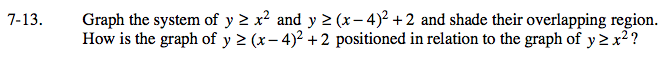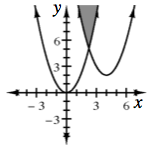### Home > A2C > Chapter 7 > Lesson 7.1.1 > Problem7-13

7-13.

Graph the system of y ≥ x2 and y ≥ (x − 4)2 + 2 and shade their overlapping region. How is the graph of y ≥ (x − 4)2 + 2 positioned in relation to the graph of yx2? Homework Help ✎Notice the position of each vertex.

The second graph is shifted 4 units to the right. How many units up is it shifted?